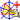Go to content
The vector subtraction AB - AC is illustrated using the "parallelogram law" to produce vector AD.

## Summary/Background

Vectors can be added together (vector addition), subtracted (vector subtraction) and multiplied by scalars (scalar multiplication). Vector multiplication is not uniquely defined, but a number of different types of products, such as the dot product and cross product can be defined for pairs of vectors.
A vector from a point A to a point B is denoted \vec{AB} , and a vector v may be denoted \bar{v} . The point A is often called the "tail" of the vector, and B is called the vector's "head." A vector with unit length is called a unit vector and often denoted using a hat, \hat{v} .
Vectors were born in the first two decades of the 19th century with the geometric representations of complex numbers. Caspar Wessel (1745--1818), Jean Robert Argand (1768--1822), Carl Friedrich Gauss (1777--1855), and at least one or two others conceived of complex numbers as points in the two-dimensional plane, i.e., as two-dimensional vectors. Mathematicians and scientists worked with and applied these new numbers in various ways; for example, Gauss made crucial use of complex numbers to prove the Fundamental Theorem of Algebra (1799). In 1837, William Rowan Hamilton (1805-1865) showed that the complex numbers could be considered abstractly as ordered pairs (a, b) of real numbers. This idea was a part of the campaign of many mathematicians, including Hamilton himself, to search for a way to extend the two-dimensional "numbers" to three dimensions; but no one was able to accomplish this, while preserving the basic algebraic properties of real and complex numbers.

## Software/Applets used on this pageUses the CabriJava interactive geometry applet. You can download the file by double-clicking on the display and then selecting the option at bottom right. You can then load it into your copy of Cabri.

## Glossary

### cross product

For vectors a and b, the cross product is the vector c whose magnitude is ab sin C, where C is the angle between the directions of the vectors, and which is perpendicular to both a and b.

### dot product

For vectors a and b, a.b=|a||b|cos C, where C is the angle between the directions of the vectors.

### geometric

A sequence where each term is obtained by multiplying the previous one by a constant.

### unit vector

A vector with magnitude equal to 1.

### vector

A mathematical object with magnitude and direction.

Full Glossary List

## This question appears in the following syllabi:

SyllabusModuleSectionTopicExam Year
AQA A-Level (UK - Pre-2017)C4Vectors2D Vector geometry-
AQA AS Maths 2017MechanicsVectorsVector Basics-
AQA AS/A2 Maths 2017MechanicsVectorsVector Basics-
CBSE XII (India)Vectors and 3-D GeometryVectorsVectors and scalars, magnitude and direction of a vector-
CCEA A-Level (NI)C4Vectors2D Vector geometry-
CIE A-Level (UK)P1Vectors2D Vector geometry-
Edexcel A-Level (UK - Pre-2017)C4Vectors2D Vector geometry-
Edexcel AS Maths 2017Pure MathsVectorsVector Basics-
Edexcel AS/A2 Maths 2017Pure MathsVectorsVector Basics-
I.B. Higher Level4Vectors2D Vector geometry-
I.B. Standard Level4Vectors2D Vector geometry-
Methods (UK)M4Vectors2D Vector geometry-
OCR A-Level (UK - Pre-2017)C4Vectors2D Vector geometry-
OCR AS Maths 2017Pure MathsVectorsVector Basics-
OCR MEI AS Maths 2017Pure MathsVectorsVector Basics-
OCR-MEI A-Level (UK - Pre-2017)C4Vectors2D Vector geometry-
Pre-Calculus (US)E1Vectors2D Vector geometry-
Pre-U A-Level (UK)6Vectors2D Vector geometry-
Scottish (Highers + Advanced)HM3Vectors2D Vector geometry-
Scottish HighersM3Vectors2D Vector geometry-
Universal (all site questions)VVectors2D Vector geometry-
WJEC A-Level (Wales)C4Vectors2D Vector geometry-